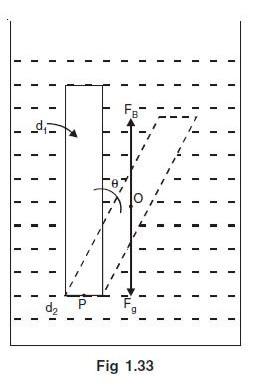# SHM in liquidA thin rod of length $L$ and area of cross section $S$ is pivoted at its lowest point P inside a stationary , homogeneous , non viscous liquid as shown in the figure.

The rod is free to rotate in a vertical plane about a horizontal axis passing through $p$. The density of the material of the rod $D_1$ is smaller than the density of liquid $D_2$. The rod is displaced by a small angle $θ$ from its equilibrium position and then released.

If the body performs Simple harmonic motion, calculate t's angular fequency $\omega$

Details and Assumptions

$D_1 = 200 \text {units}$

$D_2 = 400 \text {units}$

$L = 20 \text {cm}$

$g =10 \dfrac {m}{s^2}$

Also try this.

×

Problem Loading...

Note Loading...

Set Loading...